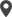Enable Dark Mode!By: Sagarika

# What is Mixin Class & How to Use Mixin Classes in Odoo 15

## Technical Odoo 15

In Python, a mixin refers to a class that provides method implementations that can be reused. Simply mixins bundle a set of methods for reuse. This feature can be used in the Odoo platform as well. This blog discusses how to use mixin classes in Odoo 15.
Consider a module for managing an Educational Organisation. This may include models for managing Students, Teachers, Parents, and many more. As a simple example considering the model's Students, Teachers and Parents there is a field that stores the Date of Birth and a field for storing the age which is computed from the Date of Birth. In such cases, the mixin class can be used. Method for computing Age from DOB can be written in the mixing class and it can be reused in other models. Now let's see this in detail.
First of all create models for managing Student, Parent, and Teacher records. Each model contains a field for setting DOB and an age field which is a computed field.
Student Model
`class Student(models.Model):   _name = "student.student"   _description = "Student"   name = fields.Char(string="Name", required=True)   partner_id = fields.Many2one('res.partner', string="Partner")   phone = fields.Char(string="Phone Number")   email = fields.Char(string="Email", required=True)   status = fields.Char(string="Status")   date = fields.Date(string="Date", default=fields.Date.today())   total_grade = fields.Float(string="Total Grade")   dob = fields.Date(string="DOB")   age = fields.Integer(string="Age", compute='_compute_age')`
Parent Model
`class Parent(models.Model):   _name = "student.parent"   _description = "Parent"   name = fields.Char(string="Name", required=True)   phone = fields.Char(string="Phone Number")   email = fields.Char(string="Email")   partner_id = fields.Many2one('res.partner', string="Partner", required=True)   date = fields.Date(string="Date", default=fields.Date.today())   student_ids = fields.Many2many('student.student', string="Students")   dob = fields.Date(string="DOB")   age = fields.Integer(string="Age", compute='_compute_age')`
Teacher Model
`class Teacher(models.Model):   _name = "teacher.teacher"   _description = "Teacher"   name = fields.Char(string="Name", required=True)   phone = fields.Char(string="Phone Number")   email = fields.Char(string="Email")   partner_id = fields.Many2one('res.partner', string="Partner", required=True)   date = fields.Date(string="Date", default=fields.Date.today())   student_ids = fields.Many2many('student.student', string="Students")   dob = fields.Date(string="DOB")   age = fields.Integer(string="Age", compute='_compute_age')`
Method used to calculate age from a date is the same in all cases. It is not a good idea to write the same compute method in every model. So define this method inside a mixin class and reuse this method in all models by simply inheriting the mixin class. Inheriting the mixin class in the model enables you to reuse the method defined inside the mixin class.
Define a mixin class for defining the computing age method.
Mixin Class
`from odoo import fieldsfrom dateutil.relativedelta import relativedeltaimport logging_logger = logging.getLogger(__name__)class GetAgeMixin:   def get_age_from_dob(self, dob):       """      :return: Returns age.      """       age = 0       today = fields.Date.today()       if dob:           age = relativedelta(today, dob).years       return age`
Here get_age_from_dob() takes dob as the method argument and returns age if there is a value in dob; else this returns 0. Now let us see how we can reuse this method inside other models. For that, inherit GetAgeMixin class in the model. Typically, a child class uses multiple inheritances to combine the mixin classes with a parent class. Hence it enables to use of all properties of the parent class. For that model, the definition has to be rewritten as follows.
`class Student(models.Model, GetAgeMixin):   _name = "student.student"   _description = "Student"`
If the mixin class is defined in the same python, you can simply use the mixin by referring to the mixin class. If it is defined in any other python file or any other module, then first you have to import mixin for using it. After inheriting the mixin class, write the compute method as shown below.
`@api.depends('dob')def _compute_age(self):   for rec in self:       rec.age = self.get_age_from_dob(rec.dob)`
Here you don't have to write the code which computes age using DOB in all models. You can call the method defined inside the mixin class for computation by passing the DOB as the parameter. Since the method returns age value you can simply assign it to the age field.
This is the way how the python mixins class works in the Odoo platform. Here described is a simple example. But when it comes to an implementation system, mixins classes will be very helpful for applying complex business logic and reusing logic in different classes.

If you need any assistance in odoo, we are online, please chat with us.

### Recent Posts

#### How To Patch Existing OWL Component in Odoo 17Calicut

Cybrosys Technologies Pvt. Ltd.
Neospace, Kinfra Techno Park
Kakkancherry, Calicut
Kerala, India - 673635London

Cybrosys Limited
Alpha House,
100 Borough High Street, London,
SE1 1LB, United KingdomKochi

Cybrosys Technologies Pvt. Ltd.
1st Floor, Thapasya Building,
Kochi, India - 682030.Bangalore

Cybrosys Techno Solutions
The Estate, 8th Floor,